# RS Aggarwal Solutions For Class 7 Maths Exercise 1D Chapter 1 Integers

The pdf of RS Aggarwal Solutions for the Exercise 1D of Class 7 Maths Chapter 1, Integers are available here. The main aim is to help students understand and crack these problems. We at BYJU’S have prepared the RS Aggarwal Solutions for Class 7 Maths wherein problems are solved step by step with detailed explanations. To cover the entire syllabus in Maths, the RS Aggarwal is a necessary material as it gives a wide range of questions that test the students’ understanding of concepts.

## Download the PDF of RS Aggarwal Solutions For Class 7 Maths Chapter 1 Integers – Exercise 1D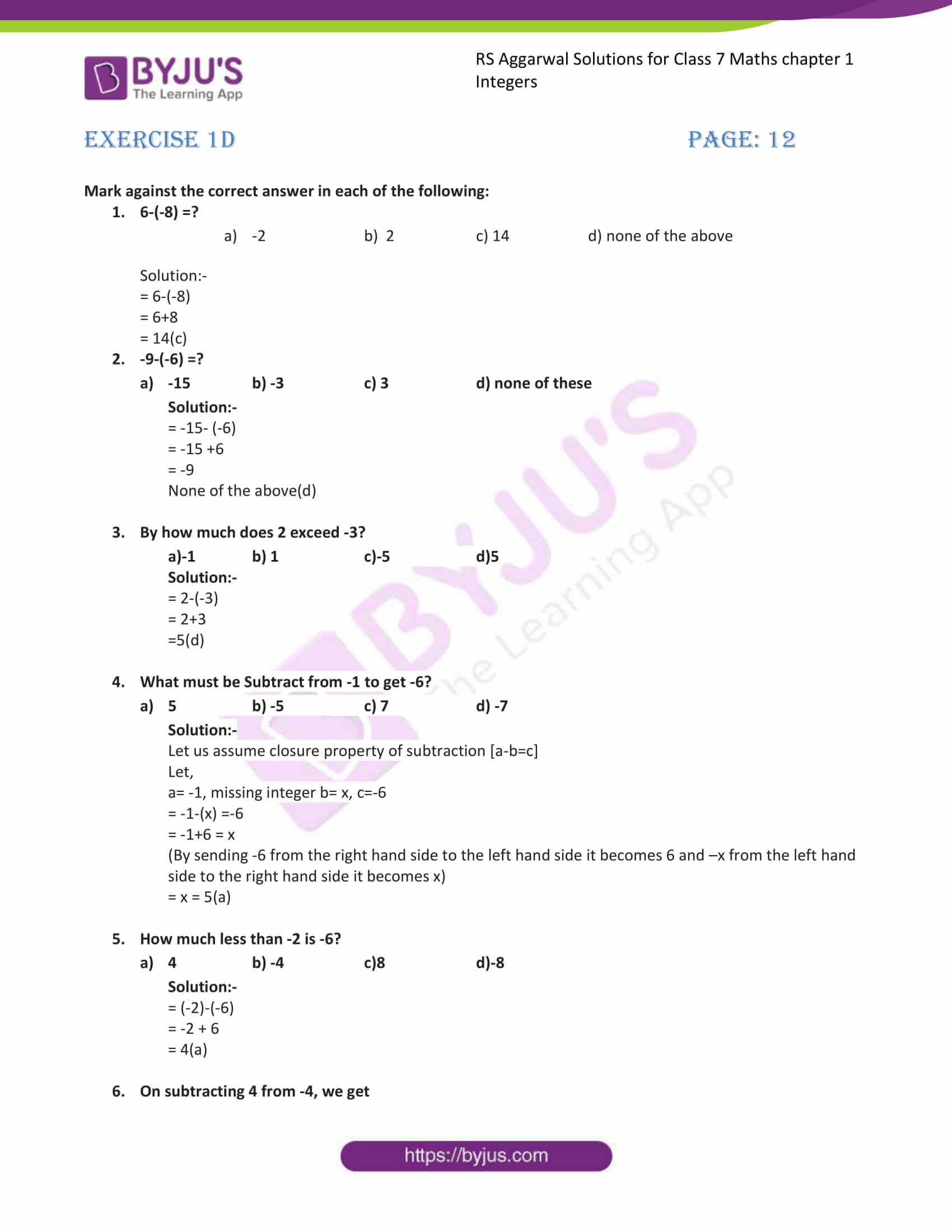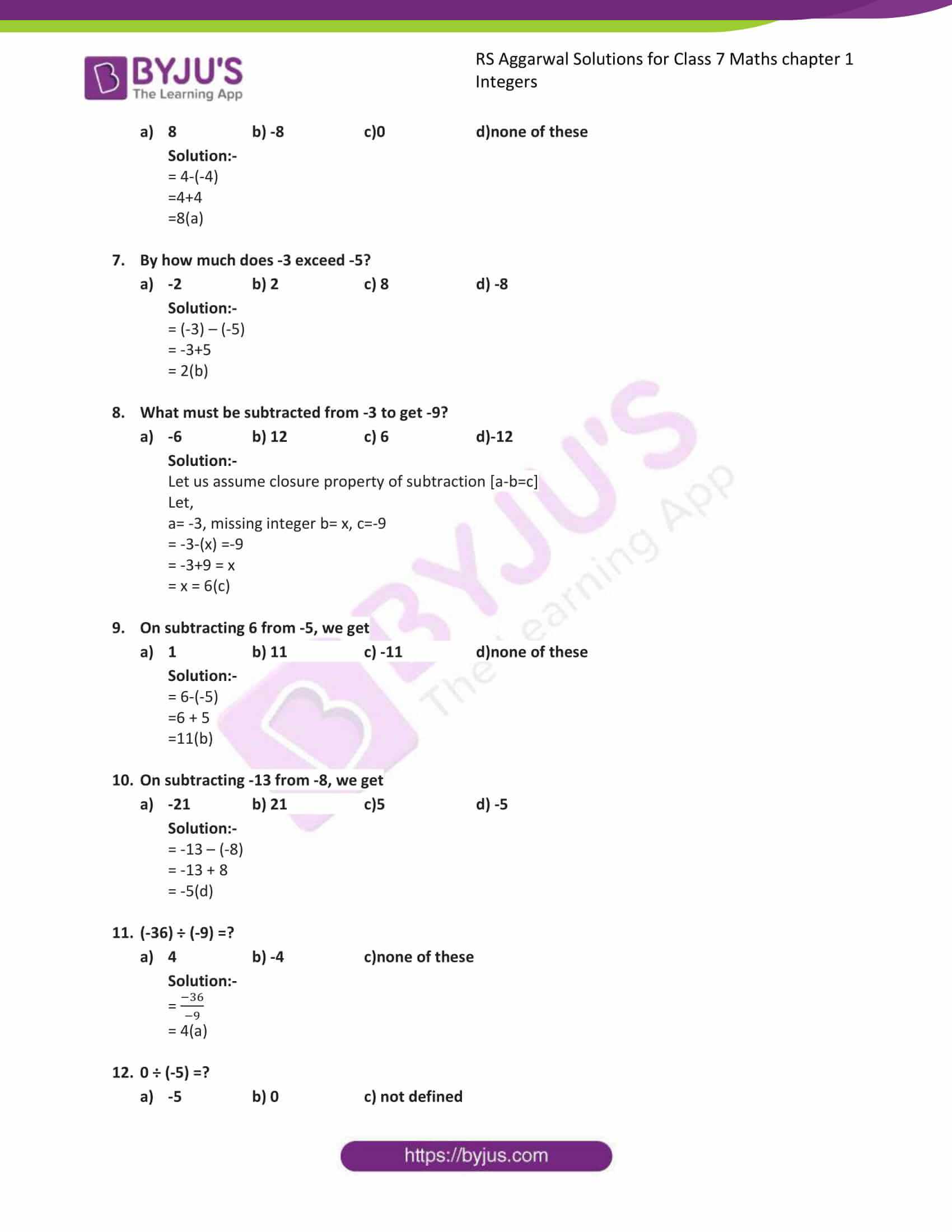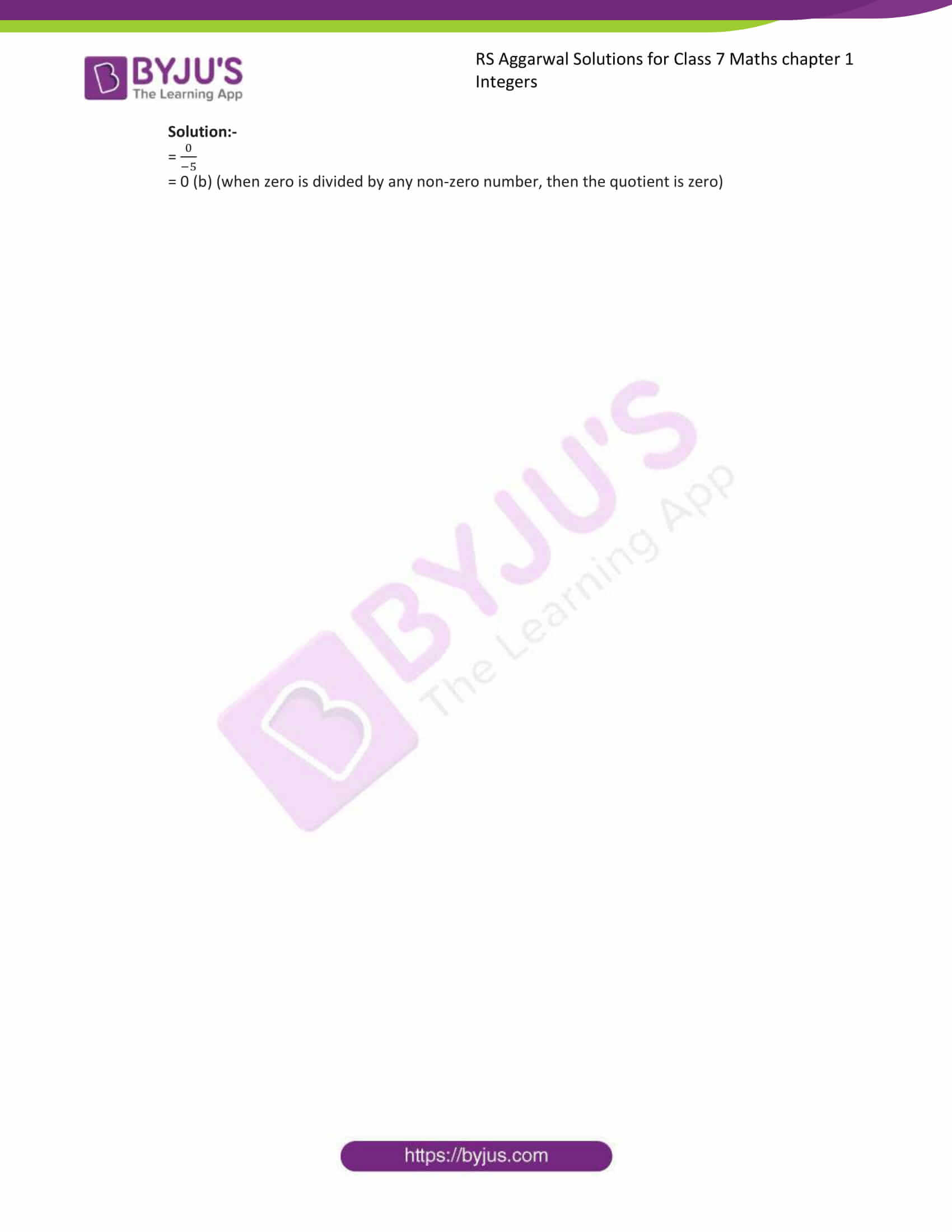### Access answers to Maths RS Aggarwal Solutions for Class 7 Chapter 1 – Integers Exercise 1D

Mark against the correct answer in each of the following: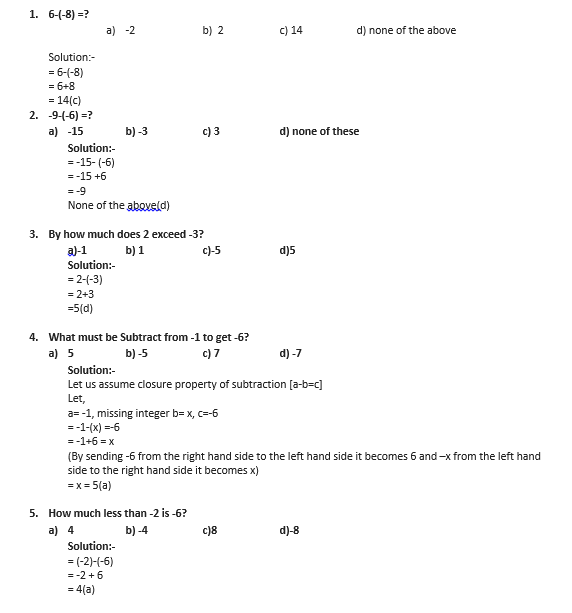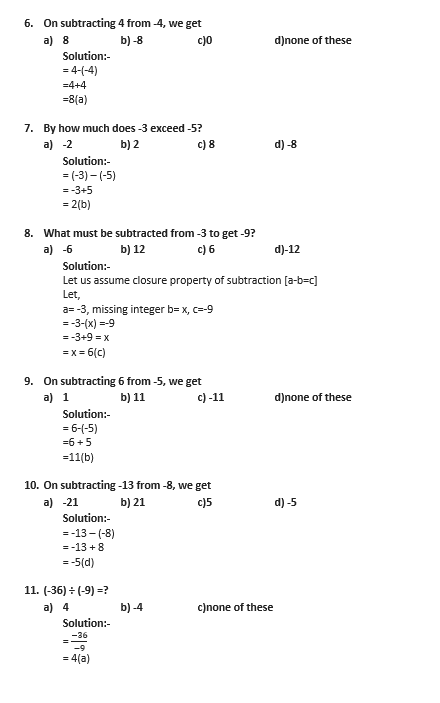12. 0 ÷ (-5) =?

a) -5 b) 0 c) not defined

Solution:-

= 0/(-5)

= 0 (b) (when zero is divided by any non-zero number, then the quotient is zero)

### RS Aggarwal Solutions for Class 7 Maths Exercise 1D Chapter – 1 Integers

RS Aggarwal Solutions For Class 7 Maths Chapter 1 Integers Exercise 1D has objective type questions. In this exercise, application-level questions are included to help students think out of the box to get the answers. The objective type questions in this exercise are based on all the topics covered in this chapter. Students are suggested to try solving the problems from RS Aggarwal Solutions book of Class 7 and then refer to these solutions to know the best way of approaching the different questions.